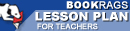Lesson Plans

# The Logic of Scientific Discovery Quiz | Eight Week Quiz E

This set of Lesson Plans consists of approximately 102 pages of tests, essay questions, lessons, and other teaching materials.
 View a FREE sampleName: _________________________ Period: ___________________

This quiz consists of 5 multiple choice and 5 short answer questions through Part II: Chapter 7, Simplicity.

## Multiple Choice Questions

1. What does the first rule of logic do?
(a) Sets a norm.
(b) States testability.
(c) Determines the type of research.
(d) Proves a scientific statement.

2. What is one approach to knowledge considered by Popper?
(a) Accidental.
(b) Common sense.
(c) Purposive.
(d) Inductive.

3. What is the reproducible effect which refutes a theory?
(a) Falsifiability.
(c) Falsification.
(d) Convention.

4. What does Popper feel is a fruitless exercise?
(a) Naming universal things.
(b) Naming singular things.
(c) Naming metaphysical things.
(d) Naming logical things.

5. What is the most significant factor when selecting a theory?
(a) The degree of testability.
(b) Previous research.
(c) Its universality.
(d) The understandability.

1. What does Popper feel is the result of adopting conditions to a hypothesis?

2. How do conventionalists define simplicity?

3. What increases with the amount of falsifiability?

4. What type of reasoning does Popper deny exists?

5. According to many theorists, what gives meaning to classes of events?

 This section contains 194 words (approx. 1 page at 300 words per page) View a FREE sample